﻿ 基于膜系统的粒子群算法 Particle Swarm Optimization Algorithm Based on Membrane System

Computer Science and Application
Vol. 09  No. 07 ( 2019 ), Article ID: 31446 , 10 pages
10.12677/CSA.2019.97158

Particle Swarm Optimization Algorithm Based on Membrane System

Nan Song, Taowei Chen, Yimin Yu, Kun Zhao

Information Institute, Yunnan University of Finance and Economics, Kunming Yunnan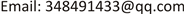Received: Jul. 5th, 2019; accepted: Jul. 19th, 2019; published: Jul. 26th, 2019ABSTRACT

Due to the particle swarm algorithm has the characteristics of easy understanding and implementation, it is widely concerned in the field of science and engineering, but its search strategy is relatively simple, making it difficult for the algorithm to obtain the Pareto frontier and easy to fall into local optimal and infinite iteration. There are still some deficiencies in the aspect of global search and convergence. Inspired by the P system theory, this paper proposes a multi-objective particle swarm optimization algorithm based on the membrane system framework to solve the multi-objective optimization problem. According to the hierarchical structure, objects and rules of P system, the particle swarm algorithm is used to implement the parallel search strategy in the base film. The non-dominated sorting and congestion distance mechanism are used in the surface film to improve the convergence speed of the algorithm, and the evolution rule is used to maintain the diversity of solution. The simulation experiment uses the KUR, ZDT series and DTLZ series test functions to test the MPSO algorithm and compare it with other multi-objective optimization algorithms, including MOPSO, dMOPSO, SMPSO, MMOPSO, MOEA/D, SPEA2, PESA2, NSGAII. The experimental results show that the new algorithm can guarantee the diversity of the solution, is fast convergence and close to the real Pareto frontier.

Keywords:Particle Swarm Optimization, Membrane System, Multi-Objective Optimization, Pareto Frontier, Non-Dominated Sorting1. 引言

2. 相关概念

2.1. 多目标优化问题

$\begin{array}{l}\text{min}\text{\hspace{0.17em}}\text{\hspace{0.17em}}\text{\hspace{0.17em}}\text{\hspace{0.17em}}\text{f}\left(x\right)=\left[{f}_{1}\left(x\right),{f}_{2}\left(x\right){,\cdots ,f}_{M}\left(x\right)\right]\\ st{.}_{\text{\hspace{0.17em}}\text{\hspace{0.17em}}\text{\hspace{0.17em}}\text{\hspace{0.17em}}\text{\hspace{0.17em}}\text{\hspace{0.17em}}h\left(x\right)=\left[{h}_{1}\left(x\right),{h}_{2}\left(x\right){,\cdots ,h}_{K}\left(x\right)\right]=0}^{\text{\hspace{0.17em}}\text{\hspace{0.17em}}\text{\hspace{0.17em}}\text{\hspace{0.17em}}\text{\hspace{0.17em}}\text{\hspace{0.17em}}g\left(x\right)=\left[{g}_{1}\left(x\right),{g}_{2}\left(x\right){,\cdots ,g}_{J}\left(x\right)\right]\ge 0}\\ \text{\hspace{0.17em}}\text{\hspace{0.17em}}\text{\hspace{0.17em}}\text{\hspace{0.17em}}\text{\hspace{0.17em}}\text{\hspace{0.17em}}\text{\hspace{0.17em}}\text{\hspace{0.17em}}\text{\hspace{0.17em}}{{x}_{i}}^{\left(L\right)}\le {x}_{i}\le {x}^{\left(U\right)},i=1,\cdots ,N\end{array}$ (1)

(1)式中 $X={\left({x}_{1},{x}_{2},\cdots ,{x}_{N}\right)}^{T}$$N$ 维决策向量，且取值在 ${{x}_{i}}^{\left(L\right)}\le {x}_{i}\le {x}^{\left(U\right)}$ 之间； $f\left(x\right)$ 定义了 $M$ 个目标函数；(1)中定义包含了 $J$ 个不等式约束 $g\left(x\right)$ 个等式约束 $h\left(x\right)$ ，约束函数 $\text{g}\left(x\right)$$h\left(x\right)$ 共同确定决策向量中 $X$ 的可行域。

2.2. 粒子群算法

$\begin{array}{l}{V}_{\text{t}+1}=\omega *{V}_{\text{t}}+{c}_{\text{1}}*rand\left(\right)*\left(pBest-{X}_{\text{t}}\right)+{c}_{\text{2}}*Rand\left(\right)*\left(gBest-{X}_{\text{t}}\right)\\ {X}_{\text{t+1}}\text{=}{X}_{\text{t}}\text{+}{V}_{\text{t+1}}\end{array}$ (2)

${V}_{\text{t}}$${V}_{\text{t+1}}$ 分别是第 $t$ 和第 $t+1$ 次飞行的速度； ${X}_{\text{t}}$${X}_{\text{t+1}}$ 分别是经过第 $t$$t+1$ 次飞行后粒子落在的位置； $\omega$ 为惯性权重(inertia weight)，描述粒子的惯性对当前速度的影响，其大小用来平衡局部和全局的搜索能力； ${c}_{1}$${c}_{2}$ 为个体最优和全局最优的学习因子； $rand\left(\right)$$Rand\left(\right)$$\left[0,1\right]$ 上相互独立的随机数； $pBest$ 是该粒子历次飞行中“最好”的位置； $\text{g}Best$ 是种群中“最好”的粒子位置。经过多次迭代，种群中的粒子会逐渐向“更好”的位置飞行，最终求得最优解。

2.3. 膜系统

2) $T\subseteq V$ 是输出字母表；

3) $C\subseteq V$ 是催化剂；

4) $\mu$ 是一个由 $m$ 个膜组成的膜结构，各个膜及其所围的区域用标号集 $H$ 表示， $H=\left\{1,2,3,\cdots ,m\right\}$ ，其中 $m$ 称为膜系统 $\prod$ 的度；

5)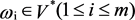表示膜结构 $\mu$ 中区域 $\text{i}$ 包含的对象多重集；

6) ${R}_{\text{i}}\left(1\le \text{i}\le m\right)$ 是关于膜系统 $\prod$ 和区域 $\mu$ 的进化规则的有限集；

7) 膜结构可等价表示为： $\prod \text{=}\left\{V,T,C,\mu ,{\omega }_{1},{\omega }_{2}{,\cdots ,\omega }_{m},{R}_{1},{R}_{1},\cdots ,{R}_{m}\right\}$

P系统是从活细胞在内部结构处理化合物的方式中抽象出来的一种计算模型 。在膜结构所定义的区域中，目标对象用字符表示，根据给定的规则实现进化。因此膜系统作为一个建模框架可以很好的与其他进化算法相结合，用来构建一个用于求解近似优化的多目标进化算法，具有易于理解、可扩展性和可编程性、固定分块性和处理离散数据的能力等优点 。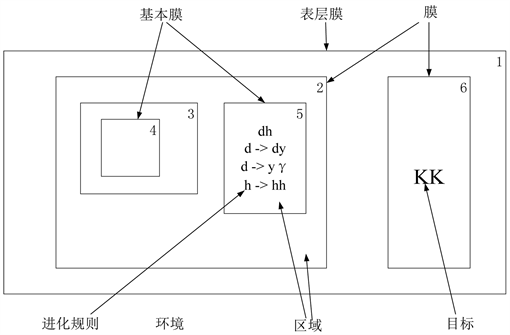Figure 1. Cell type P system structure

3. MPSO

${\text{d}}_{i}={\sum }_{m=1}^{n}\frac{{f}_{m}^{i+1}-{f}_{m}^{i-1}}{{f}_{m}^{\mathrm{max}}-{f}_{m}^{\mathrm{min}}}$ (3)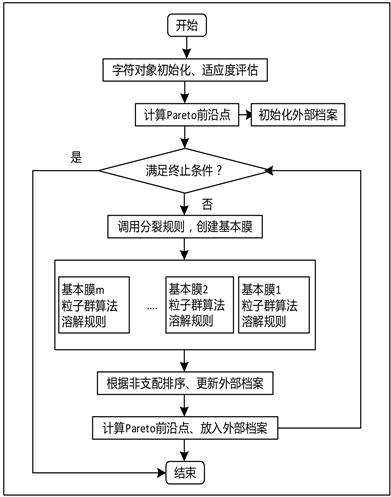Figure 2. Algorithm flowchart

${S}_{i,j}=\left({S}_{\mathrm{max},j}-{S}_{\mathrm{min},j}\right)*rand\left(\right)+{S}_{\mathrm{max},j}$ (4)

$\begin{array}{l}\omega =sort\left(\omega \right)\\ \omega =\left\{{\omega }_{1},{\omega }_{2},\cdots ,{\omega }_{m}\right\}\\ n=\frac{sizeof\left(\omega \right)}{m}\end{array}$ (5)

$\omega$ 为字符对象解集，函数 $sort\left(\right)$ 利用非支配排序返回一系列解 $\omega$ 并在解集 $\omega$ 中使用通信规则 $\left(w,in\right)$$n$ 表示每个基本膜中字符对象的数量。

4. 仿真实验

4.1. 实验设置

4.2. 二维目标实验结果分析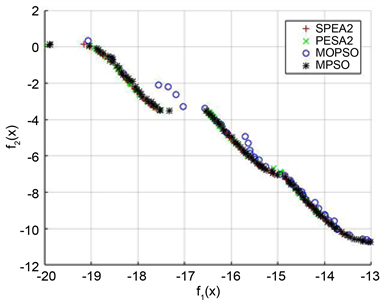Figure 3. The approximate Pareto frontier distribution point map under KURFigure 4. The approximate Pareto frontier distribution point map under ZDT1

IGD数值的高低能够反映算法的收敛性与多样性，故根据表1所示的IGD性能数据，可以看出MPSO对比于其他优化算法在KUR、ZDT3、ZDT6测试函数上相对占优，说明MPSO具有较好的收敛性、多样性以及稳定性。

4.3. 三维目标实验结果分析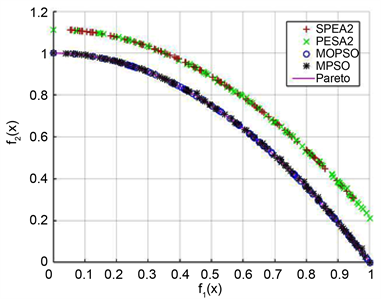Figure 5. The approximate Pareto frontier distribution point map under ZDT2Figure 6. The approximate Pareto frontier distribution point map under ZDT3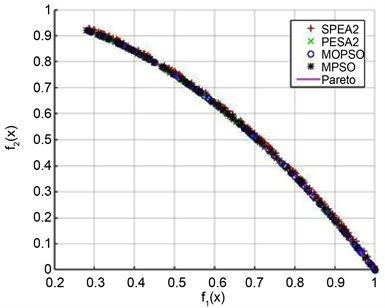Figure 7. The approximate Pareto frontier distribution point map under ZDT6Table 1. IGD performance statistics of different algorithms on five test functionsTable 2. IGD performance statistics of different algorithms on 7 test functions

ZDT2、ZDT3、ZDT6测试函数上。因此说明，PSO算法具有较好的平衡全局和局部搜索能力。

5. 结论

Particle Swarm Optimization Algorithm Based on Membrane System[J]. 计算机科学与应用, 2019, 09(07): 1406-1415. https://doi.org/10.12677/CSA.2019.97158

1. 1. Deb, K. (2001) Multi-Objective Optimization Using Evolutionary Algorithms. John Wiley & Sons, Chichester.

2. 2. 宁伟康. 进化多目标优化算法研究及其应用[D]: [博士学位论文]. 西安: 西安电子科技大学, 2018.

3. 3. Li, X. (2003) A Non-Dominated Sorting Particle Swarm Optimizer for Multi-Objective Optimization. Genetic and Evolutionary Computation, Genetic and Evolutionary Com-putation Conference, Chicago, 12-16 July 2003, 37-48. https://doi.org/10.1007/3-540-45105-6_4

4. 4. Coello, C.A.C., Pulido, G.T. and Lechuga, M.S. (2004) Handling Multiple Objec-tives with Particle Swarm Optimization. IEEE Press, Piscataway. https://doi.org/10.1109/TEVC.2004.826067

5. 5. Zhang, Q. and Li, R. (2013) Cloud Resource Scheduling Based On Improved Particle Swarm Optimization Algorithm by Membrane Computing. Computer Engineering and Applications, 49, 40-44.

6. 6. Liu, C., Han, M. and Wang, X. (2011) A Multi-Objective Evolutionary Algorithm Based on Membrane Systems. The 4th International Workshop on Advanced Computational Intelligence, Wuhan, 103-109. https://doi.org/10.1109/IWACI.2011.6159983

7. 7. Parsopoulos, K.E. and Vrahatis, M.N. (2005) Unified Particle Swarm Opti-mization for Tackling Operations Research Problems. Swarm Intelligence Symposium, Pasadena, 8-10 June 2005, 53-59.

8. 8. Hu, X. and Eberhart, R.C. (2002) Multi-Objective Optimization Using Dynamic Neighborhood Particle Swarm Optimization. Congress on Evolutionary Computation, Honolulu, 12-17 May 2002, 1677-1681.

9. 9. Dou, Z. and Gao, L. (2012) Feature Selection in Conditional Random Fields Using a Membrane Particle Swarm Optimizer. Journal of Xidian University, 39, 107-112.

10. 10. Kennedy, J. and Eber-hart, R. (1995) Particle Swarm Optimization. Proceedings of IEEE International Conference on Neural Networks, Perth, 27 Novem-ber-1 December 1995, Vol. 4, 1942-1948. https://doi.org/10.1109/ICNN.1995.488968

11. 11. Paun, G., Rozenberg, G. and Salomaa, A. (2009) Handbook of Membrane Computing. Oxford University Press, Oxford. https://doi.org/10.1007/978-3-642-11467-0

12. 12. 韩丽莎. 类细胞P系统在划分聚类中的研究[D]: [硕士学位论文]. 济南: 山东师范大学, 2015.

13. 13. 葛平平. 可满足性问题的改进型类组织P系统的求解研究[D]: [硕士学位论文]. 淮南: 安徽理工大学, 2015.

14. 14. 王康. 细胞型和神经型P系统的应用问题研究[D]: [硕士学位论文]. 成都: 西华大学, 2011.

15. 15. 张葛祥, 潘林强. 自然计算的新分支——膜计算[J]. 计算机学报, 2010, 33(2): 208-214.

16. 16. Pan, L.Q., Wang, J. and Hoogeboom, H.J. (2011) Spiking Neural P Systems with Astrocytes. Neural Computation, 24, 805-825. https://doi.org/10.1162/NECO_a_00238

17. 17. Ye, L. and Guo, P. (2016) Design a Membrane System for Solving Linear Equa-tions. Journal of Computational and Theoretical Nanoscience, 13, 1561-1568. https://doi.org/10.1166/jctn.2016.5081

18. 18. 公茂果, 焦李成, 杨咚咚, 等. 进化多目标优化算法研究[J]. 软件学报, 2009, 20(2): 271-289.